POV-Ray : Documentation : 1.3.3.3 Isosurface ObjectPOV-Ray 3.6 Documentation Online View#### 1.3.3.3 Isosurface Object

You know you have been raytracing too long when ...
... You find yourself wishing you'd paid attention in math class to all those formulae you thought you'd never have any use for in real life.
-- Jeff Lee

Isosurfaces are shapes described by mathematical functions.

In contrast to the other mathematically based shapes in POV-Ray, isosurfaces are approximated during rendering and therefore they are sometimes more difficult to handle. However, they offer many interesting possibilities, like real deformations and surface displacements

Some knowledge about mathematical functions and geometry is useful, but not necessarily required to work with isosurfaces.

##### 1.3.3.3.1 Simple functions

For the start we will choose a most simple function: `x` The value of this function is exactly the current x-coordinate.

The isosurface object takes this function as a user defined function:

```  isosurface {
function { x }
contained_by { box { -2, 2 } }
}
```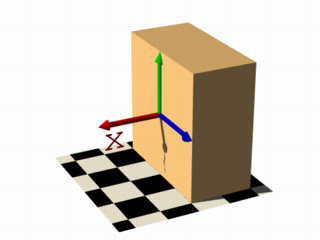the resulting shape is fairly simple: a box.

The fact that it is a box is only caused by the container object which is required for an isosurface. You can either use a box or a sphere for this purpose.

So only one side of the box is made by the function in fact. This surface is where the x-coordinate is 0 since 0 is the default threshold. There usually is no reason to change this, since it is the most common and most suggestive value, but you can specify something different by adding

` threshold 1`

to the isosurface definition.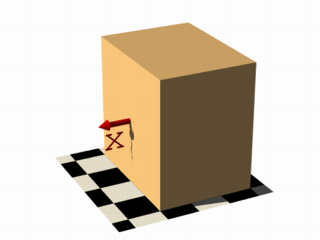As you can see, the surface is now at x-coordinate 1.

We can also remove the visible surfaces of the container object by adding the word 'open' to the isosurface definition.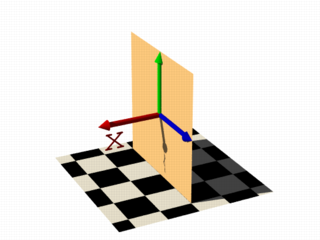For making it clearer what surfaces are the actual isosurface and what are caused by the container object, the color will be different in all the following pictures.

Now we replace the used function with something different:

` function { x+y }`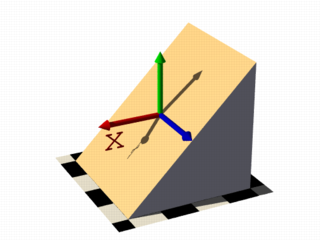` function { x+y+z }`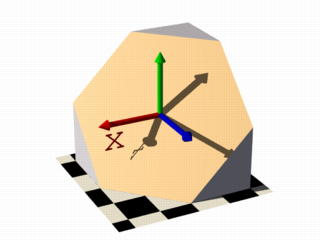Note: 'max_gradient 4' is added to the isosurface definition here, this will be explained later on.

All these functions describe planes going through the origin. The function just describes the normal vector of this plane.

##### 1.3.3.3.2 Several surfaces

The following two functions lead to identical results:

` function { abs(x)-1 }`

` function { sqrt(x*x)-1 }`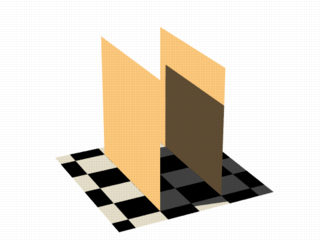You can see that there are two planes now. The reason is that both formulas have the same two solutions (where the function value is 0), namely `x=-1` and `x=1`.

We can now mix all these elements in different combinations, the results always consist of plane surfaces:

` function { abs(x)-1+y }`` function { abs(x)+abs(y)+abs(z)-2 }`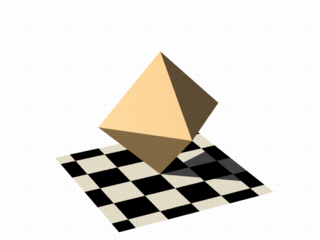##### 1.3.3.3.3 Non-linear functions

Curved surfaces of many different kinds can be achieved with non-linear functions.

` function { pow(x,2) + y }`You can see the parabolic shape caused by the square function.

To get a cylindrical surface we can use the following function.

` function { sqrt(pow(x,2) + pow(z,2)) - 1 }`

In 2 dimensions it describes a circle, since it is constant in the 3rd dimension, we get a cylinder: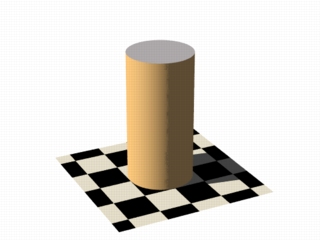It is of course not difficult to change this into a cone, we just need to add a linear component in y-direction:

` function { sqrt(pow(x,2) + pow(z,2)) + y }`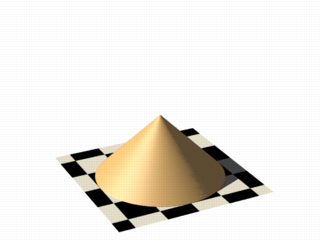And we of course can also make a sphere:

` function { sqrt(pow(x,2) + pow(y,2) + pow(z,2)) - 2 }`The `2` specifies the radius here.

##### 1.3.3.3.4 Specifying functions

As we have seen, the functions used to define the isosurface are written in the `function {...}` block.

Allowed are:

User defined functions (like equations). All float expressions and operators (see section "User-Defined Functions") which are legal in POV-Ray, can be used.
With the equation of a sphere "```x^2+y^2+z^2 = Threshold```" we get:

```isosurface {
function {pow(x,2) + pow(y,2) + pow(z,2)}
threshold Threshold
...
}
```

Functions can be declared first (see section "Declaring Functions") and then used in the isosurface.

```#declare Sphere = function {pow(x,2) + pow(y,2) + pow(z,2)}
isosurface {
function { Sphere(x,y,z) }
threshold Threshold
...
}
```

By default a function takes three parameters (x,y,z) and you do not have to explicitly specify the parameter names when declaring it.
When using the identifier, the parameters must be specified.
On the other hand, if you need more or less than three parameters when declaring a function, you also have to explicitly specify the parameter names.

```#declare Sphere = function(x,y,z,Radius) {
pow(x,2) + pow(y,2) + pow(z,2) - pow(Radius,2)
}
isosurface {
function { Sphere(x,y,z,1) }
...
}
```
##### 1.3.3.3.5 Internal functions

There are a lot of internal functions available in POV-Ray. For example a sphere could also be generated with ```function { f_sphere(x, y, z, 2) }``` These functions are declared in the `functions.inc` include file. Most of them are more complicated and it is usually faster to use them instead of a hand coded equivalent. See the complete list for details.

The following makes a torus just like POV-Ray's torus object:

```  #include "functions.inc"

isosurface {
function { f_torus(x, y, z, 1.6, 0.4) }
contained_by { box { -2, 2 } }
}
```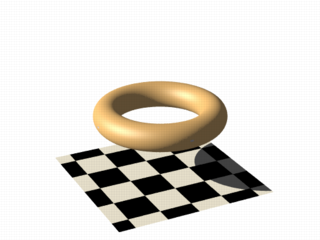The 4th and 5th parameter are the major and minor radius, just like the corresponding values in the `torus{}` object.

The parameters x, y and z are required, because it is a declared function. You can also declare functions yourself like it is explained in the reference section.

##### 1.3.3.3.6 Combining isosurface functions

We can also simulate some Constructive Solid Geometry with isosurface functions. If you do not know about CSG we suggest you have a look at "What is CSG?" or the corresponding part of the reference section first.

We will take two functions: a cylinder and a rotated box:

```  #declare fn_A = function { sqrt(pow(y,2) + pow(z,2)) - 0.8 }
#declare fn_B = function { abs(x)+abs(y)-1 }
```

If we combine them the following way, we get a "merge":

`function { min(fn_A(x, y, z), fn_B(x, y, z)) }`An "intersection" can be obtained by using `max()` instead of `min()`:

`function { max(fn_A(x, y, z), fn_B(x, y, z)) }`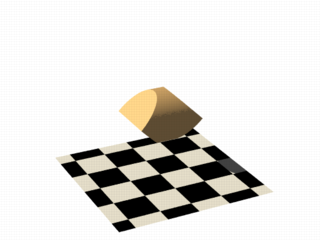Of course also "difference" is possible, we just have to add a minus (-) before the second function:

`function { max(fn_A(x, y, z), -fn_B(x, y, z)) }`Apart from basic CSG you can also obtain smooth transits between the different surfaces (like with the blob object)

```  #declare Blob_threshold=0.01;

isosurface {
function {
(1+Blob_threshold)
-pow(Blob_threshold, fn_A(x,y,z))
-pow(Blob_threshold, fn_B(x,y,z))
}
contained_by { box { -2, 2 } }
}
```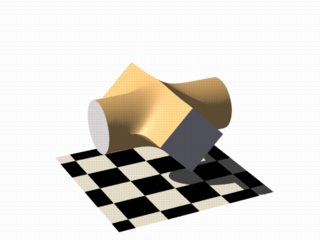The `Blob_threshold` value influences the smoothness of the transit between the shapes. a lower value leads to sharper edges.

The function for a negative blob looks like:

```function{fn_A(x,y,z) + pow(Blob_threshold,(Fn_B(x,y,z) + Strength))}
```
##### 1.3.3.3.7 Noise and pigment functions

Some of the internal functions have a random or noise-like structure

Together with the pigment functions they are one of the most powerful tools for designing isosurfaces. We can add real surface displacement to the objects rather than only normal perturbation known from the normal{} statement.

The relevant internal functions are:

• `f_noise3d(x,y,z)`
uses the noise generator specified in `global_settings{}` and generates structures like the bozo pattern.
• `f_noise_generator(x, y, z, noise_generator)`
generates noise with a specified noise generator.
• `f_ridged_mf(x, y, z, H, Lacunarity, Octaves, Offset, Gain, noise_generator)`
generates a ridged multifractal pattern.
• `f_ridge(x, y, z, Lambda, Octaves, Omega, Offset, Ridge, noise_generator)`
generates another noise with ridges.
• `f_hetero_mf(x, y, z, H, Lacunarity, Octaves, Offset, T, noise_generator)`
generates heterogenic multifractal noise.

Using pure noise3d as a function results in the following picture:

` function { f_noise3d(x, y, z)-0.5 }`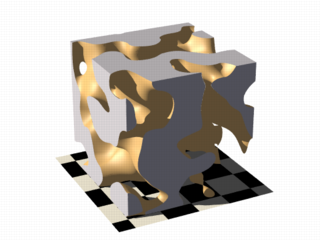Note: the `-0.5` is only there to make it match to the used threshold value of 0, the `f_noise3d` function returns values between 0 and 1.

With this and the other functions you can generate objects similar to heightfields, having the advantage that a high resolution can be achieved without high memory requirements.

` function { x + f_noise3d(0, y, z) }`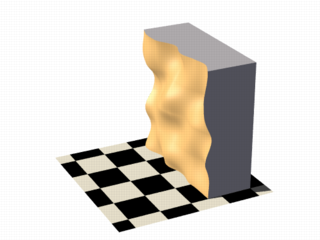The noise function can of course also be subtracted which results in an 'inverted' version:

` function { x - f_noise3d(0, y, z) }`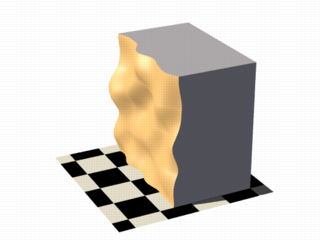In the last two pictures we added the noise function to a plane function. The x-parameter was set to 0 so the noise function is constant in x-direction. This way we achieve the typical heightfield structure.

Of course we can also add noise to any other function. If the noise function is very strong this can result in several separated surfaces.

` function { f_sphere(x, y, z, 1.2) - f_noise3d(x, y, z) }`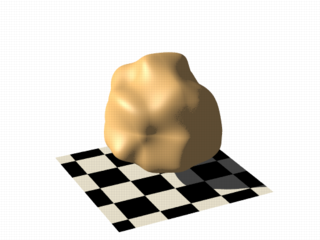This is a noise function applied to a sphere surface, we can influence the intensity of the noise by multiplying it with a factor and change the scale by multiplying the coordinate parameters:

` function { f_sphere(x, y, z, 1.6) - f_noise3d(x * 5, y * 5, z * 5) * 0.5 }`As alternative to noise functions we can also use any pigment in a function:

```  #declare fn_Pigm=function {
pigment {
agate
color_map {
[0 color rgb 0]
[1 color rgb 1]
}
}
}
```

This function is a vector function returning a (color) vector.For use in isosurface functions they must be declared first. When using the identifier, you have to specify which component of the color vector should be used. To do this, the dot notation is used: `Function(x,y,z).red`.

A color vector has five components. Supported dot types to access these components are:

• F( ).`x` | F( ).`u` | F( ).`red`
• to get the red value of the color vector
• F( ).`y` | F( ).`v` | F( ).`green`
• to get the green value of the color vector
• F( ).`z` | F( ).`blue`
• to get the blue value of the color vector
• F( ).`filter` | F( ).`t`
• to get the filter value of the color vector
• F( ).`transmit`
• to get the transmit value of the color vector
• F( ).`gray`
• to get the gray value of the color vector
• gray value = Red*29.7% + Green*58.9% + Blue*11.4%
• F( ).`hf`
• to get the height_field value of the color vector
• hf value = (Red + Green/255)*0.996093
• the .hf operator is experimental and will generate a warning.

` function { f_sphere(x, y, z, 1.6)-fn_Pigm(x/2, y/2, z/2).gray*0.5 }`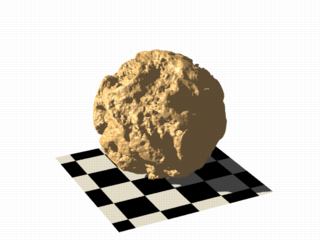There are quite a lot of things possible with pigment functions, but you probably have recognized that this renders quite slow.

##### 1.3.3.3.8 Conditional directives and loops

Conditional directives are allowed in functions:

```#declare Rough = yes;
#include "functions.inc"
isosurface {
function { y #if(Rough=1)-f_noise3d(x/0.5,y/0.3,z/0.4)*0.8 #end }
...
}
```

Loops can also be used in functions:

```#include "functions.inc"
#declare Thr = 1/1000;
#declare Offset = 1.5;
#declare Scale = 1.2;
#declare TrSph = function { f_sphere(x-Offset,y,z,0.7*Scale) }

function {
(1-Thr)
#declare A = 0;
#while (A<8)
-pow(Thr, TrSph(x*cos(A*Ang) + y*sin(A*Ang),
y*cos(A*Ang) -x*sin(A*Ang), z) )
#declare A=A+1;
#end
}
```

Note: The loops and conditionals are evaluated at parse time, not at render time.

##### 1.3.3.3.9 Transformations on functions

Transforming an isosurface object is done like transforming any POV-Ray object. Simply use the object modifiers (scale, translate, rotate, ...).

However, when you want to transform functions within the contained_by object, you have to substitute parameters in the functions.

The results seem inverted to what you would normally expect. Here is an explanation:
Take a Sphere(x,y,z). We know it sits at the origin because x=0. When we want it at x=2 (translating 2 units to the right) we need to write the second equation in the same form: x-2=0
Now that both equations equal 0, we can replace parameter x with x-2
So our Sphere(x-2, y,z) moves two units to the right.

Let's scale our Sphere 0.5 in the y direction. Default size is y=1 (one unit). We want y=0.5.
To get this equation in the same form as the first one, we have to multiply both sides by two. y*2 = 0.5*2, which gives y*2=1
Now we can replace the y parameter in our sphere: Sphere(x, y*2, z). This squishes the y-size of the sphere by half.
Well, this is the general idea of substitutions.

Here is an overview of some useful substitutions:
Using a declared object P(x,y,z)

Scale
scale x : replace "`x`" with "`x/scale`" (idem other parameters)

`scale x*2   gives    P(x/2,y,z)`

Scale Infinitely
scale x infinitely : replace "`x`" with "`0`" (idem other parameters)

`scale y infinitely   gives    P(x,0,z)`

Translate
translate x : replace "`x`" with "`x - translation`" (idem other parameters)

`translate z*3   gives    P(x,y,z-3)`

Shear
shear in XY-plane : replace "`x`" with "```x + y*tan(radians(Angle))```" (idem other parameters)

`shear 45 degrees left   gives    P(x+y*tan(radians(45)), y, z)`

Rotate

Note: these rotation substitutions work like normal POV-rotations: they already compensate for the inverse working

rotate around X
: replace "`y`" with "```z*sin(radians(Angle)) + y*cos(radians(Angle))```"
: replace "`z`" with "```z*cos(radians(Angle)) - y*sin(radians(Angle))```"

rotate around Y
: replace "`x`" with "```x*cos(radians(Angle)) - z*sin(radians(Angle))```"
: replace "`z`" with "```x*sin(radians(Angle)) + z*cos(radians(Angle))```"

rotate around Z
: replace "`x`" with "```x*cos(radians(Angle)) + y*sin(radians(Angle))```"
: replace "`y`" with "```-x*sin(radians(Angle)) + y*cos(radians(Angle)) ```"

```  rotate z*75   gives:
```

Flip
flip X - Y : replace "`x`" with "`y`" and replace "`y`" with "`-x`"

flip Y - Z : replace "`y`" with "`z`" and replace "`z`" with "`-y`"

flip X - Z : replace "`x`" with "`-z`" and replace "`z`" with "`x`"

`flip x and y   gives    P(y, -x, z)`

Twist
twist N turns/unit around `X`
: replace "`y`" with "`z*sin(x*2*pi*N) + y*cos(x*2*pi*N)`"
: replace "`z`" with "```z*cos(x*2*pi*N) - y*sin(x*2*pi*N)```"

##### 1.3.3.3.10 Improving Isosurface Speed

To optimize the approximation of the isosurface and to get maximum rendering speed it is important to adapt certain values;

`accuracy`

The accuracy value influences how accurate the surface geometry is calculated. Lower values lead to a more precise, but slower result. The default value of `0.001` is fairly low. We used this value in all the previous samples, but often you can raise this quite a lot and thereby make things faster.

`max_gradient`

For finding the actual surface it is important for POV-Ray to know the maximum gradient of the function, meaning how fast the function value changes. We can specify a value with the `max_gradient` keyword. Lower max_gradient values lead to faster rendering, but if the specified value is below the actual maximum gradient of the function, there can be holes or other artefacts in the surface.

For the same reason functions with infinite gradient should not be used. This applies for pigment functions with brick or checker pattern for example. You should also be careful when using `select()` in isosurface functions because of this.

If the real maximum gradient differs too much from the specified value POV-Ray prints a warning together with the found maximum gradient. It is usually sufficient to use this number for the `max_gradient` parameter to get fast and correct results.

POV-Ray can also dynamically change the `max_gradient` when you specify `evaluate` with 3 parameters the isosurface definition. Concerning the details on this and other things see the evaluate in the reference section.

`contained_by`

Make sure your `contained_by` 'object' fits as tightly as possible. An oversized container can sky-rocket the render time.
When the container has a lot of empty space around the actual isosurface, POV-Ray has to do a lot of superfluous sampling: especially with complex functions this can become very time consuming. On top of this, the `max_gradient` needed to get a proper surface will also increase rapidly (almost proportional to the oversize!).
You could use a transparent copy of the container (using exactly the same transformations) to check how it fits. Getting the `min_extent` and `max_extent` of the `isosurface` is not useful because it only gives the extent of the container and not of the actual isosurface.